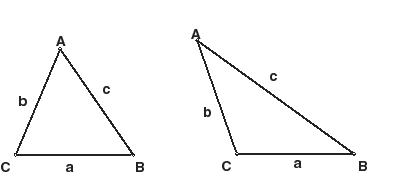# Proof by Contradiction

A proof by contradiction also known as an indirect proof, establishes the truth of a given proposition by the supposition that it is false and the subsequent drawing of a conclusion that is contradictory to something that is proven to be true. That is, the supposition thatis false followed necessarily by the conclusionfrom not-, whereis false, which implies thatis true.

The proposition is proved by assuming that the opposite of the proposition is true and showing that this assumption is false, thereby implying that the proposition must be true

In other words The basic idea is to assume that the statement we want to prove is false, and then show that this assumption leads to nonsense. We are then led to conclude that we were wrong to assume the statement was false, so the statement must be true.

Examples:

#### Irrationality of the square root of 2

A classic proof by contradiction from mathematics is the proof that the square root of 2 is irrational. If it were rational, it could be expressed as a fraction a/b in lowest terms, where a and b are integers, at least one of which is odd. But if a/b = √2, then a2 = 2b2. Therefore a2 must be even. Because the square of an odd number is odd, that in turn implies that a is even. This means that b must be odd because a/b is in lowest terms.

On the other hand, if a is even, then a2 is a multiple of 4. If a2 is a multiple of 4 and a2 = 2b2, then 2b2 is a multiple of 4, and therefore b2 is even, and so is b.

So b is odd and even, a contradiction. Therefore the initial assumption—that √2 can be expressed as a fraction—must be false.

## The Converse of a Theorem

The Converse of “If P, Then Q” is the assertion “If Q, Then P”. For example, the converse of “If it is my car, it’s red” is “If the car is red, then its mine.” It should be clear from this example that there is no guarantee that the converse of a true stement is true.

Proof by Contradiction is often the most natural way to prove the converse of an already proved theorem.

## The Converse of the Pythagorean Theorem

The Pythagorean Theorem tells us that in a right triangle, there is a simple relation between the two leg lengths (a and b) and the hypotenuse length, c, of a right triangle: a2 + b2 = c2. Perhaps you don’t know that the converse is also true.

The Converse of the Pythagporean Theorem. If the (nonzero) three side lengths of a triangle–a, b and c–satisfy the relation a2 + b2 = c2, then the triangle is a right triangle. (Assume the Pythagorean Theorem has already been proved.)

Proof. (Proof by Contradiction.) Suppose the triangle is not a right triangle. Label the vertices A, B and C as pictured. (There are two possibilites for the measure of angle C: less than 90 degrees (left picture) or greater than 90 degrees (right picture).)Erect a perpendicular line segment CD as pictured below.By the Pythagorean Theorem, BD2 = a2 + b2 = c2, and so BD = c. Thus we have isosceles triangles ACD and ABD. It follows that we have congruent angles CDA = CAD and BDA = DAB. But this contradicts the apparent inequalities (see picture) BDA < CDA = CAD < DAB (left picture) or DAB < CAD = CDA < BDA (right picture).